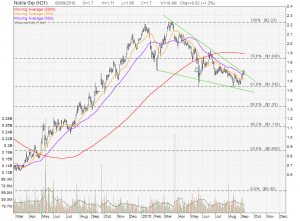## Noble Group: Breaking Out?

Noble group rebounced from the 61.8% Fibonacci Retracement support at \$1.542 and looks like breaking out from the Falling Wedge. Noble is currently testing the wedge resistance at about \$1.70. If Noble breaks out and stays above this wedge resistance (and also 20D & 50D MA supports), the stock may reach the price target of \$2.20.### This Post Has 11 Comments

1.Hi Marubozu,

How can I calculate the intrinsic value of Noble Group using DCF method in this case as the Cash from Operating Activities is currently negative?

2.Marubozu

Roy, normally I only calculate intrinsic value for my value investing. One of the value investing criteria for stock selection is the company must have consistent growth in sales revenue, net profit and net operating cash flow year over year. In this case, you can’t calculate intrinsic value for Noble group since the net operating cash flow is negative. You may see whether you can calculate the intrinsic value using EPS or use PE ratio.

Marubozu

3.I made the calculation using Discounted EPS & Discounted Equity w/ Dividend method and come out with two different numbers (\$1.44 vs \$1.19) having the same discount factor.
I am in a dilemma as to which one to follow. Hope to hear your opinion.

P.S. I do not know how to use P/E ratio in determining the intrinsic value.

4.Marubozu

Discounted EPS Model:
Rolling EPS = \$0.10196
EPS 10 year Growth Rate = 26% (cap at 15%)
Note: Annual EPS growth rate is not consistent year over year.
Beta = 1.14
Discount Rate = 7%
Intrinsic Value = \$1.55

PE Model:
Rolling EPS = \$0.10196
Average of Past 3 years PE (12.4, 13.16, 26.8) = 17.4 (for benchmarking purpose)
Intrinsic Value (PE @ 15 fair value)= PE * EPS = 15 * \$0.10196 = \$1.53.
Intrinsic Value = (PE @ 17 three years average) = 17.4 * \$0.10196 = \$1.77

==> Use the most conversative intrinsic value base on different calculation = \$1.53

Using PEG Ratio:
Current PE = Today closing price / Rolling EPS = \$1.75 / \$0.10196 = 17.16

PEG Ratio = Current PE / EPS Growth Rate = 17.16 / 15 > 1 (Over Value)

Conclusion: Noble Group is OVERVALUE!

5.Hi Marubozu,
Thank you very much for the information.

6.David

Hi,

I am an amateur and…
I was just wondering how did you derive the rolling eps here? I am confused here.

7.Marubozu

Rolling PE is getting the most recent 4 quarters EPS as the base on calculation. This is a better reflection of the valuation of a stock than using the past Financial Year’s EPS to do the PE calculation.

8.David

Hi,
So if we get the most recent 4 quarters, that will be Q3 and Q4 from 2009 and Q1 and Q2 from 2010. How do i calculate EPS? I look at the website and it gives this formula, net earnings/outstanding shares and i look at most websites, it gives the same thing. I am not sure if i am in the right direction. Can you guide me on the calculation portion?

9.Marubozu

David, you just need to add up the EPS for 4 quarters. Shareinvestor has rolling EPS calculated for you but you need to pay for the subscription…. nothing is free in Singapore..

10.Hi,

May I know what method do you guys use to calculate intrinsic value? I just simply cannot get it right.

Or do you subscribe to Shareinvestor to get the value?

I think noble is a good stock to keep an eye on right now .

11.Marubozu

Welltee,
You are not able to use DCF to calculate the Intrinsic value because the net operating cash flow is negative. You may use PE ratio or Discounted EPS model to calculate the intrinsic value. However, pls watch out for the “creative accounting” because the EPS is growing YoY but not the net operating cashflow.

Take note that Noble Group is not meeting Adam’s 7 steps / 10 steps Value Investing criteria.

Sharesinvestor does not provide the intrinsic value and we need to calculate by ourselves.

Have an awesome day!

Marubozu